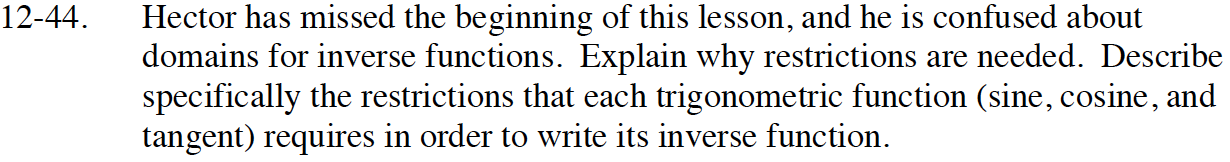### Home > INT3 > Chapter Ch12 > Lesson 12.1.3 > Problem12-44

12-44.

Hector has missed the beginning of this lesson, and he is confused about domains for inverse functions. Explain why restrictions are needed. Describe specifically the restrictions that each trigonometric function (sine, cosine, and tangent) requires in order to write its inverse function. Homework Help ✎The restrictions are needed so that the inverses will be functions.
Look back at the problems from the beginning of the lesson.
Do any of those problems help you to answer the rest of this problem?